### IMO Shortlist 2005 problem G5

Kvaliteta:
Avg: 0.0
Težina:
Avg: 8.0
Let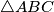$\triangle ABC$ be an acute-angled triangle with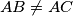$AB \not= AC$. Let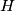$H$ be the orthocenter of triangle$ABC$, and let$M$ be the midpoint of the side$BC$. Let$D$ be a point on the side$AB$ and$E$ a point on the side$AC$ such that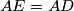$AE=AD$ and the points$D$,$H$,$E$ are on the same line. Prove that the line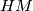$HM$ is perpendicular to the common chord of the circumscribed circles of triangle$\triangle ABC$ and triangle$\triangle ADE$.
Source: Međunarodna matematička olimpijada, shortlist 2005